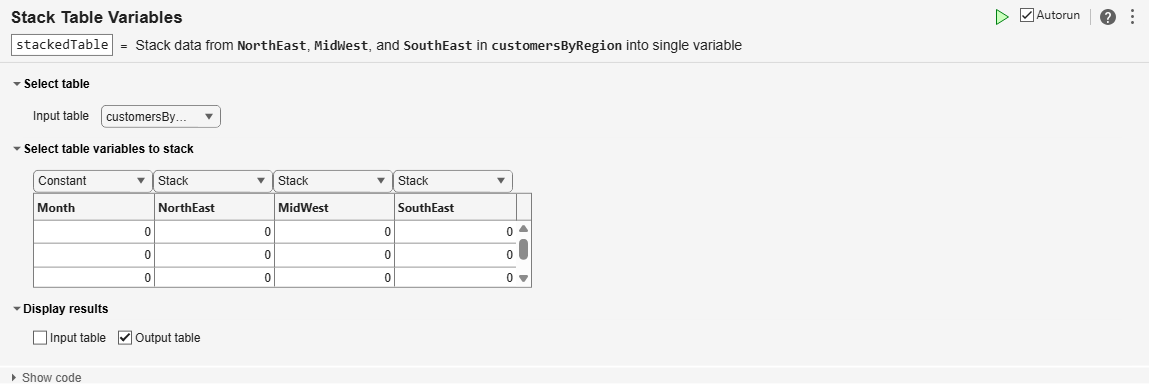# Stack Table Variables

Combine values from multiple table variables into one table variable in the Live Editor

## Description

The Stack Table Variables task lets you interactively stack values from multiple table or timetable variables into one variable. The task automatically generates MATLAB® code for your live script.

To add the Stack Table Variables task to a live script in the MATLAB Editor:

• On the Live Editor tab, select Task > Stack Table Variables.

• In a code block in the script, type a relevant keyword, such as `stack` or `table`. Select Stack Table Variables from the suggested command completions.

## Examples

expand all

This example shows how to stack variables by using the Stack Table Variables Live Editor task. It takes values from multiple variables in an input table, and combines them into one variable in an output table.

First, load a table that contains estimated influenza rates along the East Coast of the United States. The table has three variables with rates for the Northeast (`NE`), Mid-Atlantic (`MidAtl`), and Southeast (`SE`) regions. (These data come from the Google Flu Trends project, since discontinued.)

`load fluByRegion`Stack the flu rate data so that the flu rates from the `NE`, `MidAtl`, and `SE` variables are in one variable in the output table.

• To copy values from the `Month` variable to rows of the output table, select `Constant` from the drop-down list above it in the task.

• To combine the values from the `NE`, `MidAtl`, and `SE` variables by stacking them into one variable of the output, select `Stack` for each variable.

The name of the stacked variable in the output comes from combining the input variable names.

The task also stacks the names of the input variables into a new variable, named `NE_MidAtl_SE_Indicator`. In this example, the names of regions are the names of variables in the input table. Those names become data values in a new variable that indicates the regions for the flu rate data.Give the variables of the output table more meaningful names. To rename table variables, use the `renamevars` function.

```load fluRateOriginalNames fluRate = renamevars(stackedTable,["NE_MidAtl_SE_Indicator" "NE_MidAtl_SE"],["Region" "Flu Rate"]);```## Parameters

Specify the name of the input table or timetable timetables from the lists of all the nonempty tables and timetables that are in the workspace.

Specify the action to take for each variable in the input table or timetable. When stacking variables, there are three possible actions:

• Constant — Copy the variable to the output table or timetable. Replicate its values where needed in rows of the output.

• Stack — Stack the values from the variable into one variable of the output. For example, if you choose to stack three variables, all their values go into one variable of the output.

• Discard — Exclude the variable and its values from the output.